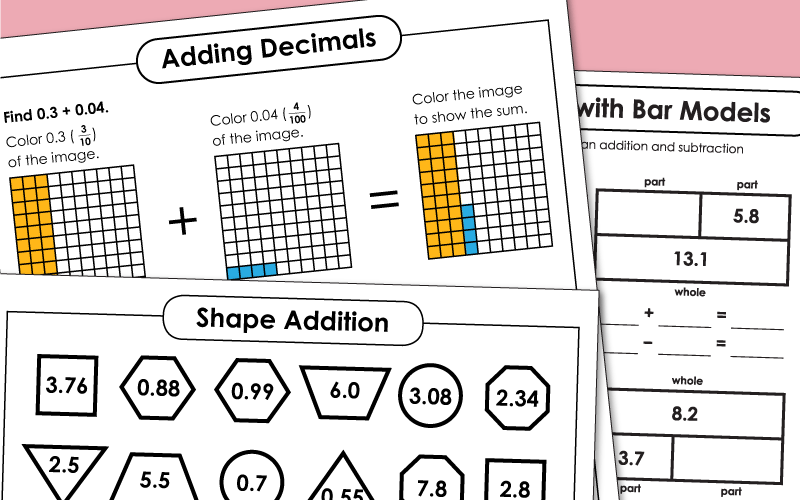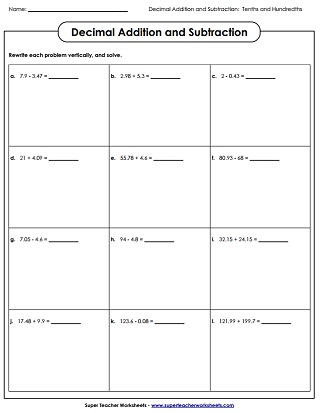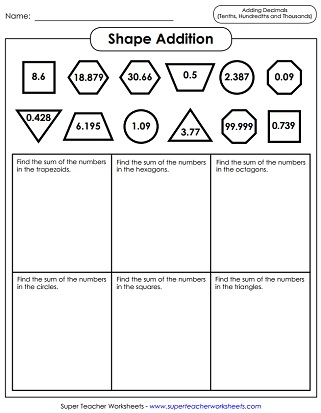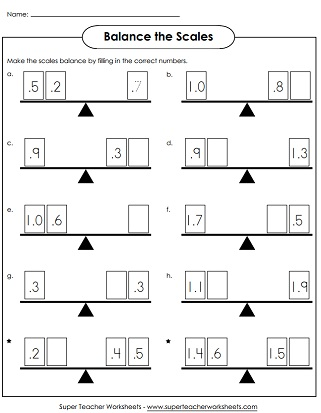# Decimal Addition & Subtraction Worksheets

Add and subtract decimals with tenths, hundredths, and thousandths place values.## Tenths

Solve twelve decimal addition problems. All problems contain whole numbers or decimals with a tenths place only. Students write each problem vertically and find the sums.
This worksheet contains a dozen subtraction problems. Students re-write each problem vertically and solve. All problems contain whole numbers or decimals with a tenths place.
This printable includes a mix of addition and subtraction problems for students to solve. Each problem needs to be re-written vertically and students must line up the columns properly.
Balance each scale by adding and subtracting basic decimal numbers.
Match up shapes with decimal numbers, then add them together.
These decimal addition problems were not solved correctly. Explain the mistakes you see. Then solve to find the correct sum.
Look very carefully at these subtraction (tenths) problems and search for errors in the solutions.
Practice decimal operations including addition and subtraction to tenths using bar models in this printable math activity.
Using bar models, students will find the missing value and then write an addition and subtraction number sentence for each one.
Complete several different types of math problems with this worksheet.

## Hundredths

Shade the correct portions of each block illustration to show the given decimal. Then add them together. Includes tenths and hundredths.
On this worksheet students are given two decimal addends for each problem. They re-write them vertically and solve. All numbers are whole numbers, or are decimals which contain a tenths or hundredths place.
Solve a dozen subtraction problems with decimal quantities. Problems must be re-written vertically to ensure students understand the place value system.
This worksheet contains a mixture of addition and subtraction problems with decimal numbers. Requires students to re-write each horizontal problem in vertical notation.
Use this worksheet to help students review and practice decimal addition and subtraction.
Find pairs of matching polygons. Then add the decimal numbers printed inside of them.
Complete each input-outbox table. All questions require students to count by quarters (example: 2.0, 2.25, 2.5, 2.75, 3.0, 3.25, and so on).
These decimal addition problems (hundredths) have errors. Examine the work carefully and determine where the solver made mistakes.
This page has subtraction problems that have already been solved. Unfortunately, there are mistakes in the solutions. Can your students find and explain the errors?
Rewrite each decimal addition or subtraction problem vertically on the graph paper, then solve. The grid paper will help students line up columns correctly.
Practice decimal operations with addition and subtraction to hundredths using bar models.
Using bar models, students will practice adding and subtracting to hundredths in this printable math activity.

## Thousandths

This page contains a dozen decimal addition problems with numbers that include a thousandths place. Students re-write each problem vertically and solve.
Use this worksheet to help students practice decimal addition and subtraction with three decimal places.
At the top of the page, students are shown a dozen shapes with decimal numbers in them. Students add numbers in congruent shapes. For example: Find the sum of the numbers in the hexagons.
Students will practice adding and subtracting to the thousandths with bar models in this printable math activity.
Students will practice decimal operations to the thousandths using bar models. They will find the missing value for each bar model. Then they will write an addition or subtraction number sentence for each.
Decimal Worksheets

Worksheets for introducing decimal concepts, comparing decimals, and ordering decimals.

Multiplying Decimals

Solve multiplication problems that have decimal factors.

Dividing Decimals

Subtracting Money

## Pictures of Our PrintablesMy Account
Site Information